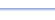Program: TRIRT

TRIRT

• Finds the third side length of a right triangle, given the other two side lengths. (Pythagorean Theorem shortcut!)
• Finds the remaining two sides lengths of a   45-45-90 triangle, given any one side length (45-45-90 Formula shortcut!)
• Finds the remaining two sides lengths of a   30-60-90 triangle, given any one side length (30-60-90 Formula shortcut!)

Useful for:

• SAT
• ACT
• Geometry
• Precalculus
• Trigonometry
• Calculus

See full list of programsExample 2

Q: Find the value of x:

Solution:

Run TRIRT.  How?

Select choice 3 for
'30-60-90'.

Choose 2 because
the given side is
the leg that is
opposite the 60°
angle.

Enter:
LEG OPP 60 = 5
The program reports:

Interpretation: